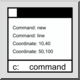## Command Line

Toolbar / Icon:Menu: View > Command Line
Shortcut: G, M
Commands: gm

### Description

The command line of QCAD allows you to start commands, enter coordinates or enter values such as distances or radii.

### Entering Coordinates

Absolute coordinates are entered in the format "x,y":

40,5

Relative coordinates are entered in the format "@x,y":

@10,6

Absolute polar coordinates are entered in the format "distance<angle":

10<30

Relative polar coordinates are entered in the format "@distance<angle":

@10<45

When entering coordinates or values, mathematical expressions may be used to calculate a coordinate based on known values. For example the coordinate 10,5 may also be entered as:

5+5,30/6

### Calculator

The command line can also be used as a calculator. To do this, enter a mathematical expression proceeded by an equal sign:

=3+4
7

Variables may be used to store values:

=a=5+6
11
=a/2
5.5

### Mathematical Expressions

Mathematical constants available are:

PI, LN2, LN10, LOG2E, LOG10E, SQRT1_2, SQRT2

Mathematical functions available are:

abs, ceil, floor, exp, log, max, min, pow, sqrt, random, round, rad2deg, deg2rad, sin, cos, tan, asin, acos, atan, atan2, log10, log1p, log2, sign, cosh, sinh, tanh, acosh, asinh, atanh, expm1, hypot, cbrt, trunc

Most of these constants and functions are standard ECMAScript (JavaScript) functions and documented online. In standard ECMAScript, these functions are part of the Math class so the function abs would have to written as Math.abs. In the QCAD command line, you can leave away the Math. part for convenience. Trigonometric functions (sin, cos, tan, asin, acos, atan, atan2) accept or return angles in degrees. If you prefer the radian versions of these functions, use the original Math. functions instead.

The functions rad2deg and deg2rad can be used to convert angles between radian and degrees.